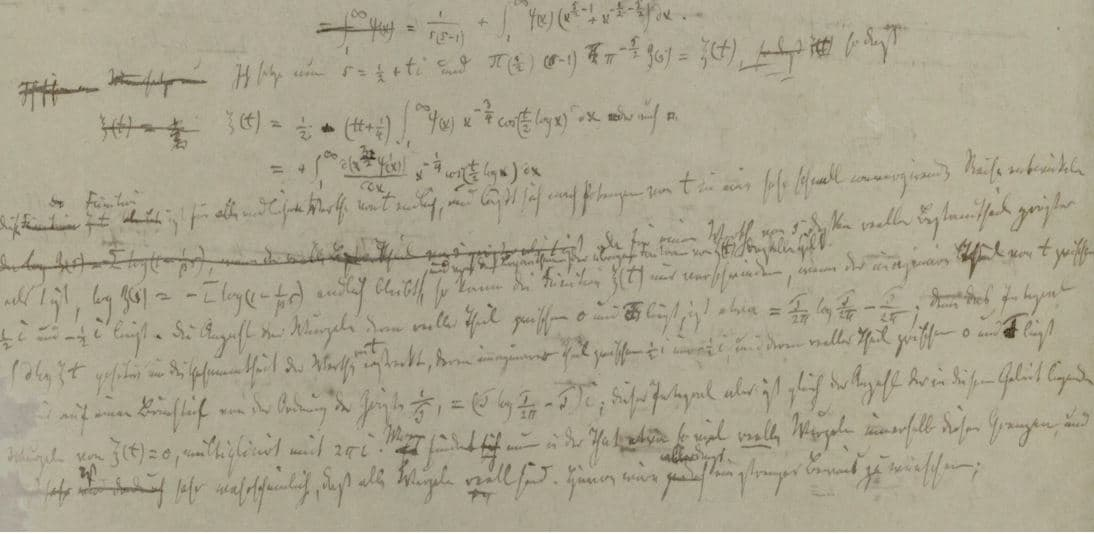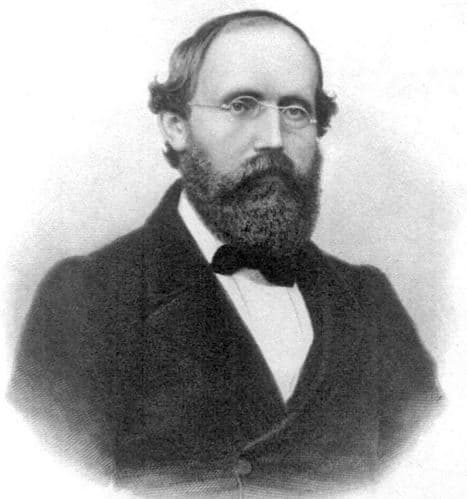# Riemann Hypothesis maths puzzle solved, claims Nigerian professor

The Riemann Hypothesis, a maths conundrum that has mystified scholars for over 150 years, has been solved, claims Dr. Opeyemi Enoch, a professor at the Federal University in Oye Ekiti, Nigeria.

The university where Dr. Enoch teaches said in a statement:

“Dr. Enoch first investigated and then established the claims of Riemann. He went on to consider and to correct the misconceptions that were communicated by mathematicians in the past generations, thus paving way for his solutions and proofs to be established.”

“He also showed how other problems of this kind can be formulated and obtained the matrix that Hilbert and Poly predicted will give these undiscovered solutions. He revealed how these solutions are applicable in cryptography, quantum information science and in quantum computers.”The Riemann Hypothesis, written by Riemann in 1859. (Image: claymath.org. Credit: The State and University Library at Göttingen)

The BBC reports that Dr. Enoch claims he was able to find a solution for a problem first proposed by the influential German mathematician Bernhard Riemann (1826-1866), who made lasting and revolutionary contributions to analysis, differential geometry and number theory.

Dr. Enoch, born in Kogi State, who has previously worked on mathematical models for generating electricity from ocean bodies, thunder and sound, could win a \$1 million prize if his claim is confirmed.

The Riemann Hypothesis

This explanation is aimed at non-mathematical readers, and therefore will miss out on much of what makes the Riemann hypothesis so interesting.

It is based on an observation Riemann made about the equation: all the equation’s values that make it go to zero seem to lie on the exact same line.

‘So what?’ you might think. But for mathematicians it is extremely interesting, because these values keep coming up in the most crazy complicated places like number theory and quantum mechanics.

Most importantly, the Riemann Hypothesis is very closely linked to prime numbers, something mathematics scholars do not understand too well.Will Dr. Enoch get \$1 million for solving one of the Millennium Prize Problems? (Image: BBC)

The Riemann Hypothesis is famous because for the past 150 years nobody has been able to solve it. This is extremely rare in maths – in the majority of cases, theories are either proved or disproved fairly quickly.

The Riemann hypothesis is also one of the seven Clay Mathematics Institute’s Millennium Prize Problems – six (including the Riemann hypothesis) remain unsolved.

1. Yang–Mills and Mass Gap.

2. Riemann Hypothesis.

3. P vs NP Problem.

4. Navier–Stokes Equation.

5. Hodge Conjecture.

6. Poincaré Conjecture.

7. Birch and Swinnerton-Dyer Conjecture.A photograph of Georg Friedrich Bernhard Riemann taken in 1863. (Image: wikipedia)

The Clay Mathematics Institute says the following regarding the Riemann Hypothesis:

“The prime number theorem determines the average distribution of the primes. The Riemann hypothesis tells us about the deviation from the average. Formulated in Riemann’s 1859 paper, it asserts that all the ‘non-obvious’ zeros of the zeta function are complex numbers with real part 1/2.”

As a matter of policy, the Clay Mathematics Institute says it never comments on solutions to the Millennium Problems.

Video – The Riemann Hypothesis

In this video, Professor Edward Frenkel, a mathematics professor at the University of California, Berkeley, talks about the Riemann Hypothesis.